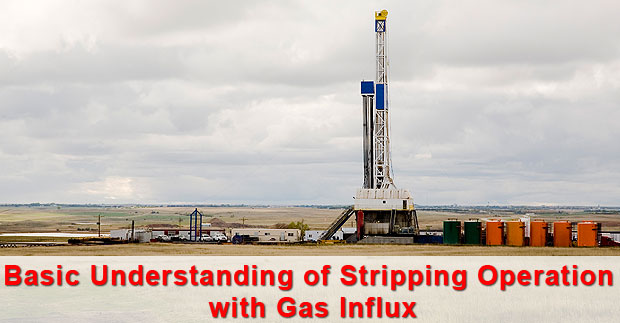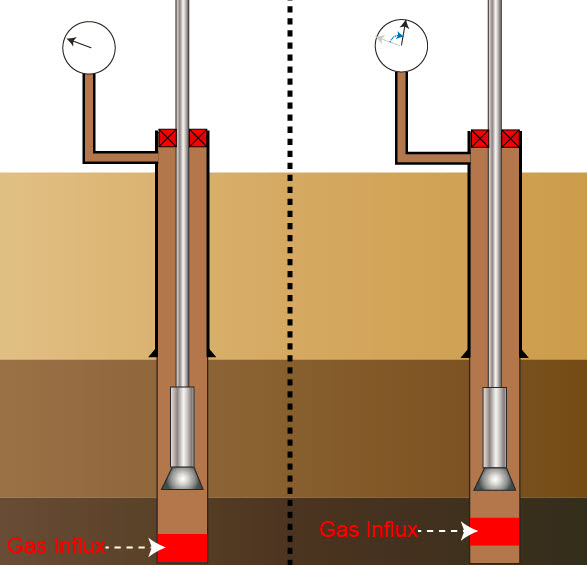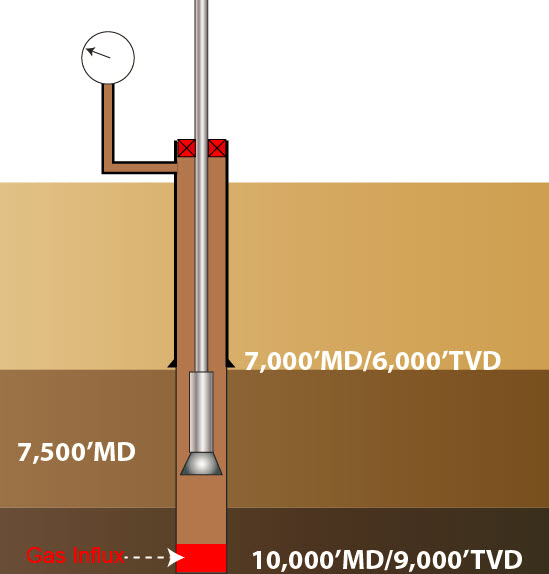# Basic Understanding of Stripping Operation Well Control with Gas Influx

In the previous articles, we discuss about the stripping operation and techniques with non-migratable kicks as oil and water kick. This article will focus on the stripping operation with gas influx and we are going to describe some additional considerations that you need to account for. Gas kick is different from the liquid kicks because gas can naturally migrate. The gas migration affects the stripping operation because increase in casing pressure due to gas migration must be taken into considerations.Gas migration can increase both surface and bottom hole pressure. If pressure increased by gas migration is not handled properly, the well can be fractured and it results in bad complications while performing well control operation.Figure 1 – Gas Migration Increases Wellbore Pressure

We will need to apply volumetric well control into the stripping operation so the procedure is called “Stripping with Volumetric Control”. This procedure will account for both pressure increased by gas migration and pipe displacement.

There are 3 figures that you need to determine before starting the operation;

Safety Factor – It is the small overbalance pressure to prevent you to be accidentally in underbalanced condition.

Pressure Increment – It is a pressure change for each step for bleeding off.

Mud Increment – It is the mud volume equivalent to Pressure Increment.

We will go through into detailed of the stripping with volumetric control procedure in a next topic.

The critical part of this operation is when the drillstring penetrates into the gas kick because height of gas increased because the annular profile changes. The will result in reduction of hydrostatic pressure. The adjustment must be made in order to compensate for loss of hydrostatic pressure. Additionally, when the drillstring penetrates into the gas kick, a new mud increment, which is based on the calculation of annular volume between ID of casing or wellbore and OD of drillstring, must be used.

With gas in the wellbore, it is quite complicated for time estimation when the drill string will penetrate the gas kick because of gas migration. You must do two calculations in order to accurately estimate the time when the drill string will penetrate the gas kick.

The first step – This is to determine gas migration rate.

Gas migration rate (ft/hr) = ∆SICP ÷ (0.052 x MW x ∆T)

Where;

∆SICP = change in casing pressure, psi

MW = mud weight in hole, ppg

∆T = Time interval of casing pressure change, hr

The second step – The time can be calculated by using the following relationship:

T = (Dgas– Dbit) ÷ (GMR + SS)

Where;

T =Time to penetrate the gas kick, hrs

Dgas = Gas kick depth, ft

Dbit = Bit depth, ft

GMR = Gas migration rate, ft/hr

SS = Stripping speed, ft/fr

Warning – This calculation may not be accurate because the calculation above is based on the known location of gas kick and the gas bubble is all together in one gas kick. If the gas is swabbed, the location of gas kick is possibly at the bottom of the bit.  Gas migration rate may change due to wellbore temperature vs depth. For the practical stand point, you may need to add safety factor which will compensate the decrease in hydrostatic and you use the conservative fluid increment by using the capacity factor around the drillstring.

Please see the following example for the calculation.

Determine gas migration rate and time to penetrate the gas kick based on the following information.

• Shoe depth = 7,000’MD/6,000’TVD
• Hole depth = 10,000’MD/9,000’TVD
• MW = 12.0 ppg
• Bit depth = 7,500 ft
• 5” DP, 19.5 ppf
• 5” DC = 800 ft as BHA
• Pit gain = 35 bbl
• Hole size = 8.5 inch
• Casing ID = 8.835 inch
• SICP = SIDP = 250 psi
• Surface pressure increases to 500 psi in 30 minutes due to gas migration.
• Average stripping speed = 200 ft/hrFigure 3 – Well Diagram for This Example

Solution

Determine gas migration rate (ft/hr)

Gas migration rate (ft/hr) = ∆SICP ÷ (0.052 x MW x ∆T)

Gas migration rate (ft/hr) = (500 – 250) ÷ (0.052 x 12 x 0.5)

Gas migration rate (ft/hr) = 801 ft/hr

Determine length of 35 bbl gas kick in 8.5” hole and top of gas kick

Length (ft) = Kick volume (bbl) ÷ Hole capacity (bbl/ft)

Hole capacity (bbl/ft) = 8.52 ÷ 1029.4 = 0.0702 bbl/ft

Length (ft) = 35 ÷ 0.0702 = 499 ft

It means that top of kick is about 499 ft from the bottom.

Top of gas kick = 10,000 – 499 = 9,501 ft

Determine time to penetrate kick

T = (Dgas– Dbit) ÷ (GMR + SS)

T = (9,501– 7,500) ÷ (801 + 200)

T = 2 hrs

Based on the given information, it will take a total of 2 hours to penetrate the gas kick.

Share the joy